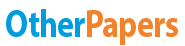# Introducing one New Product

Essay by   •  September 24, 2012  •  Essay  •  522 Words (3 Pages)  •  1,295 Views

## Essay Preview: Introducing one New Product

Report this essay
Page 1 of 3

I work for SD LLC which manufactures toys. Now SD LLC is considering introducing one new product. There are two alternatives to choose: Product A and Product B. Both A and B can be produced by present facilities. Both products require an increase in annual fixed cost of the same amount. Traditional C-V-P analysis is now being used in SD LLC. The chief executive asked me to help him to choose one product. After reading "COST-VOLUME-PROFIT ANALYSIS UNDER CONDITIONS OF UNCERTAINTY", I submitted this report.

The purpose of the article is to suggest combining probability analysis with traditional C-V-P analysis to make it a more useful tool in analyzing decision problems under uncertainty. The paper finds that traditional C-V-P analysis does not include adjustments for risk and uncertainty may, in any given instance, severely limit its usefulness. While probability analysis combined with C-V-P allows a comparison of the risk involved in each alternative, as well as a comparison of relative breakeven points and expected profits. Given the firm's attitude toward and willingness to assume risk, a probability dimension added to C-V-P analysis would be useful.

The author used the normal distribution in the C-V-P analysis. There were four fundamental relationships used in the analysis: 1. the selling price per unit; 2. the variable cost per unit; 3. the total fixed cost; and 4. the expected sales volume of each product. The above-mentioned four variables were all subjectively estimated. I believe this will make the analysis less reliable. The author would better use historical data on similar products to estimate the four variables. Moreover, the author assumed the four variables are independent and so that no correlation exists between events of the four different variables. I totally disagree with this assumption. It is obviously that there is a strong correlation between the selling price per unit and the expected sales volume. So the values of the respective covariances should be taken into account in the computational procedure.

As for my company, I believe we should use the techniques discussed in "COST-VOLUME-PROFIT ANALYSIS UNDER CONDITIONS OF UNCERTAINTY" to aid our decision making. Because traditional C-V-P analysis does not take account of the relative risk of various alternatives, while the techniques preserve the traditional analysis but also add risk as another important decision factor. If we still use traditional C-V-P analysis, we cannot compare the risk involved in each product, which maybe mislead our decision making and cause a huge loss. By applying the techniques, we can improve our decision process and control the probability of losses. To apply the techniques, we should use historical data to estimate the selling price per unit; the variable cost per unit; the total fixed cost; and the expected sales volume of each product. Then we use probability analysis combined with C-V-P to compare

...

...